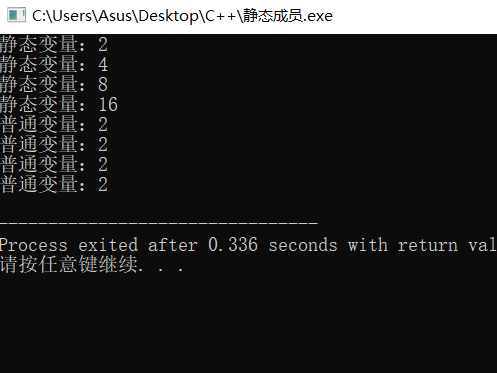# C++静态成员静态函数，语法+示例，超详细！！

2023-09-24 13 0

### 静态局部变量

``````//特殊之处的举例说明#include<iostream>
using namespace std;
void Static()
{static int n = 1;n *= 2;cout<<"静态变量："<<n<<endl;
}
void Auto()
{int n = 1;n *= 2;cout<<"普通变量："<<n<<endl;
}
int main()
{Static();Static();Static();Static();Auto();Auto();Auto();Auto();return 0;
}
``````### 类的静态成员

#### 静态成员变量的声明

``````static 数据类型 成员变量名;
``````

``````#include<iostream>
using namespace std;
class Data
{public:int n;static int m;	//静态成员变量 类内声明
};
int Data::m=0;		//类外初始化
int main()
{Data d1,d2;return 0;
}//d1.n 与 d2.n 是两个不同的变量//d1.m 与 d2.m 是同一个变量
``````

#### 静态成员变量的初始化

``````数据类型 类名::静态成员变量 = 初始值;#include<iostream>
using namespace std;
class Data
{public:int n;static int m;	//静态成员变量 类内声明
};int Data::m=0;					//类外初始化int main()
{Data d1,d2;return 0;
}
``````

### 静态成员函数

#### 静态成员函数的声明

``````static 返回值类型函数名(参数表)//在书写静态成员函数的代码时，不必写static
``````
``````#include<iostream>
using namespace std;
class Student
{int id;float score;static float sum;	//总分 static int count;	//计数public:Student(int i,int s):id(i),score(s)		//定义构造函数{count++;} void total();				//总分	static float average(); //平均数 			//静态成员函数的声明
};float Student::sum = 0;		//静态成员变量的初始化
int Student::count = 0; void Student::total()
{sum += score;
}float Student::average()		//静态成员函数
{if(count)return sum/count;
}int main()
{Student stu = {Student(1,10) , Student(2,20) , Student(3,30)};for(int i=0;i<3;i++)stu[i].total();cout<<"平均分是："<<Student::average();		//不需要用对象，因为这是共有的return 0;
}
``````

this指针 ×